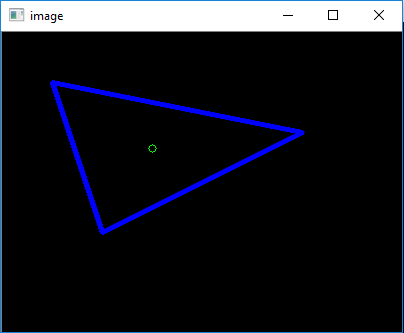# Draw a triangle with centroid using OpenCV

Prerequisite: Geometric shapes using OpenCV

Given three vertices of a triangle, write a Python program to find the centroid of the triangle and then draw the triangle with its centroid on a black window using OpenCV.

Examples:

Input: (100, 200) (50, 50) (300, 100)
Output: (150, 116)


Libraries Needed:

OpenCV
Numpy


Approach:
Create a black window with three color channels with resolution 400 x 300. Draw three lines which are passing through the given points using the inbuilt line function of the OpenCV. It will create a triangle on the black window. Find the centroid of the triangle using the following simple formula.Draw this centroid on the black window using circle function of OpenCV with zero thickness.

Below is the implementation of the above approach:

 # Python3 code to draw a triangle and find centroid     # importing libraries  import numpy as np  import cv2     # Width and height of the black window   width = 400 height = 300    # Create a black window of 400 x 300  img = np.zeros((height, width, 3), np.uint8)     # Three vertices(tuples) of the triangle   p1 = (100, 200)  p2 = (50, 50)  p3 = (300, 100)     # Drawing the triangle with the help of lines  #  on the black window With given points   # cv2.line is the inbuilt function in opencv library  cv2.line(img, p1, p2, (255, 0, 0), 3)  cv2.line(img, p2, p3, (255, 0, 0), 3)  cv2.line(img, p1, p3, (255, 0, 0), 3)     # finding centroid using the following formula  # (X, Y) = (x1 + x2 + x3//3, y1 + y2 + y3//3)   centroid = ((p1+p2+p3)//3, (p1+p2+p3)//3)     # Drawing the centroid on the window    cv2.circle(img, centroid, 4, (0, 255, 0))     # image is the title of the window  cv2.imshow("image", img)  cv2.waitKey(0)

Output:

(150, 116)Whether you're preparing for your first job interview or aiming to upskill in this ever-evolving tech landscape, GeeksforGeeks Courses are your key to success. We provide top-quality content at affordable prices, all geared towards accelerating your growth in a time-bound manner. Join the millions we've already empowered, and we're here to do the same for you. Don't miss out - check it out now!

Previous
Next